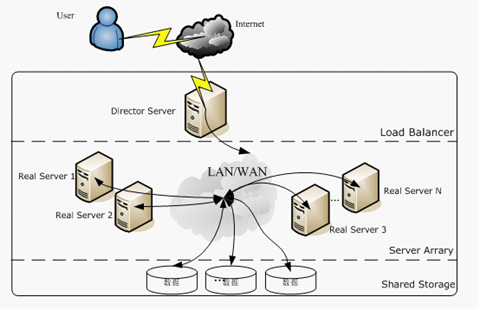# 机器学习算法之岭回归、Lasso回归和ElasticNet回归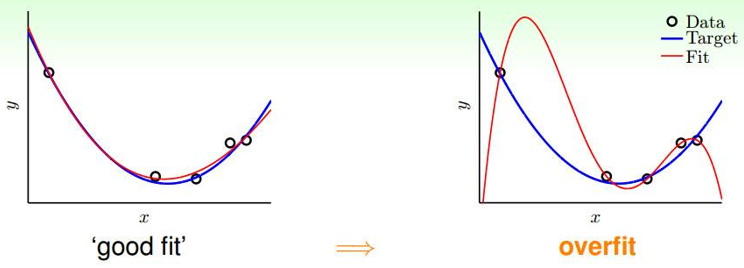## 正则化

Lasso：使用L1-norm正则

$$J(\theta)=\frac{1}{2}\sum_{i}^{m}(y^{(i)}-\theta ^Tx^{(i)})^2+\lambda \sum_{j}^{n}|\theta_j|$$

Ridge：使用L2-norm正则

$$J(\theta)=\frac{1}{2}\sum_{i}^{m}(y^{(i)}-\theta ^Tx^{(i)})^2+\lambda \sum_{j}^{n}\theta_j^2$$

ElasticNet：结合l1-norm、l2-norm进行正则

$$J(\theta)=\frac{1}{2}\sum_{i}^{m}(y^{(i)}-\theta ^Tx^{(i)})^2+\lambda (\rho\sum_{j}^{n}|\theta_j|+(1-\rho)\sum_{j}^{n}\theta_j^2)$$

1. 正则化的目的：防止过拟合
2. 正则化的本质：约束（限制）要优化的参数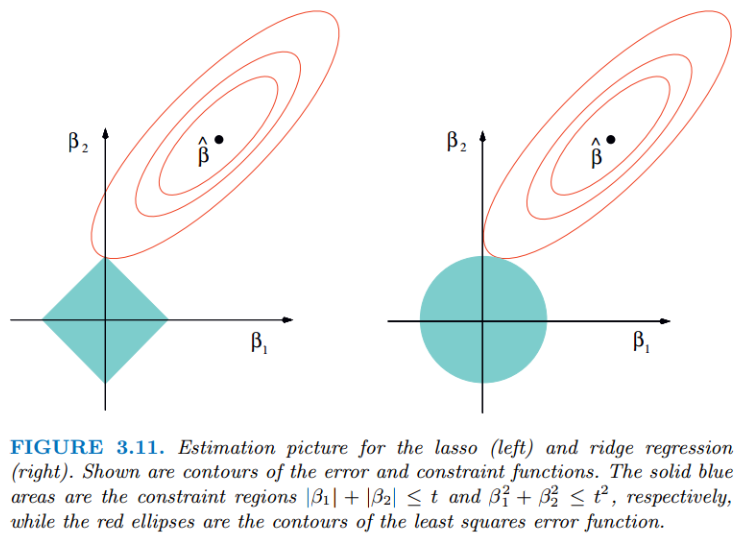1、没有约束项时。模型参数$\beta _1$，$\beta _2$已经经过标准化。残差平方和RSS可以表示为$\beta _1$，$\beta _2$的一个二次函数，数学上可以用一个抛物面表示。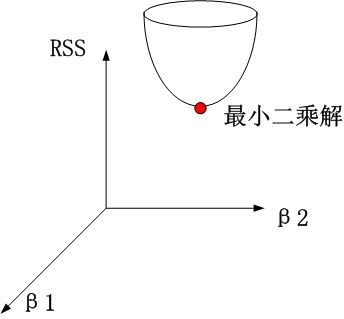2、岭回归时。约束项为$\beta _1^2 + \beta _2^2 \leq t$，对应着投影为$\beta _1$，$\beta _2$平面上的一个圆，即下图中的圆柱。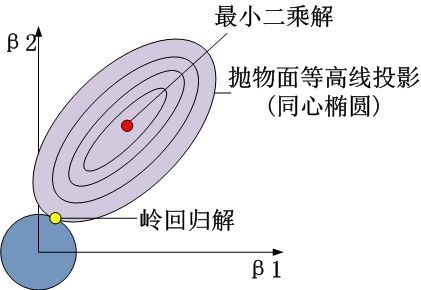## 使用Scikit-Learn进行岭回归、Lasso回归和ElasticNet回归

### 岭回归

$$L(\bar{w})=\left \| X\bar{w} -\bar{y}\right \|_2^2 + \alpha \left \| \bar{w} \right \|_2^2$$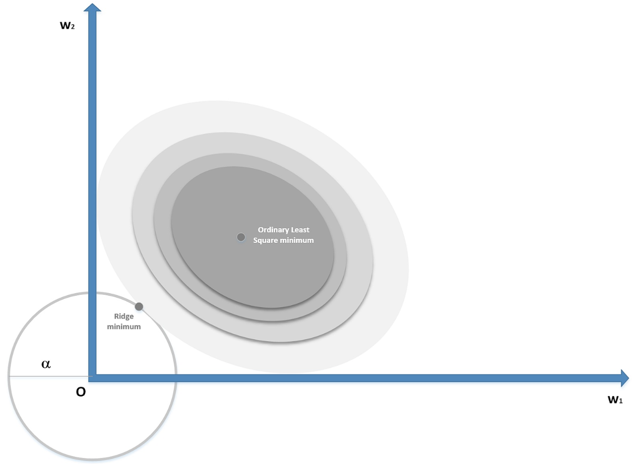from sklearn.linear_model import Ridge
from sklearn.model_selection import train_test_split

X = boston.data
y = boston.target

# 把数据分为训练数据集和测试数据集(20%数据作为测试数据集）
X_train, X_test, y_train, y_test = train_test_split(X, y, test_size=0.2, random_state=3)

model = Ridge(alpha=0.01, normalize=True)
model.fit(X_train, y_train)

train_score = model.score(X_train, y_train)  # 模型对训练样本得准确性
test_score = model.score(X_test, y_test)  # 模型对测试集的准确性
print(train_score)
print(test_score)


from sklearn.linear_model import RidgeCV
from sklearn.model_selection import train_test_split

X = boston.data
y = boston.target

# 把数据分为训练数据集和测试数据集(20%数据作为测试数据集）
X_train, X_test, y_train, y_test = train_test_split(X, y, test_size=0.2, random_state=3)

model = RidgeCV(alphas=[1.0, 0.5, 0.1, 0.05, 0.01, 0.005, 0.001, 0.0005, 0.0001], normalize=True)
model.fit(X_train, y_train)

print(model.alpha_)

### Lasso回归

Lasso回归加入w的L1范数作为惩罚项，以确定系数中的数目较多的无用项（零值）：

$$L(\bar{w})=\frac{1}{2n}\left \| X\bar{w} -\bar{y}\right \|_2^2 + \alpha \left \| \bar{w} \right \|_1$$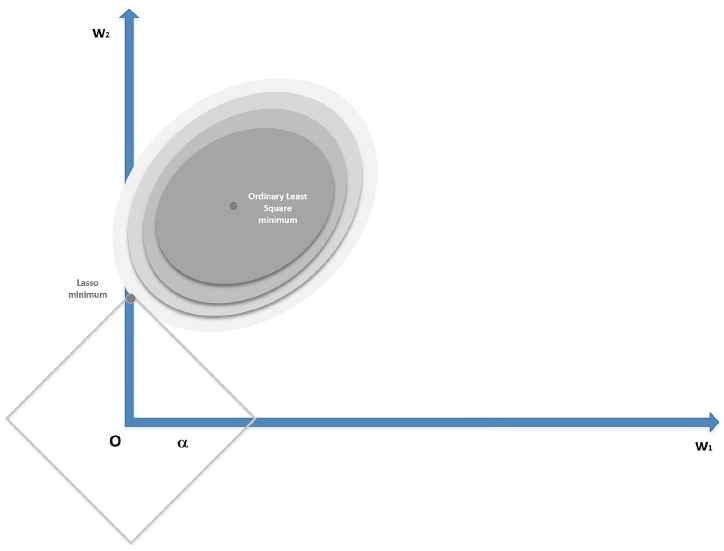### ElasticNet回归

ElasticNet将Lasso和Ridge组成一个具有两种惩罚因素的单一模型：一个与L1范数成比例，另外一个与L2范数成比例。使用这种方式方法所得到的模型就像纯粹的Lasso回归一样稀疏，但同时具有与岭回归提供的一样的正则化能力。它的损失函数是：

$$L(\bar{w})=\frac{1}{2n}\left \| X\bar{w} -\bar{y}\right \|_2^2 + \alpha \beta \left \| \bar{w} \right \|_1 + \frac{\alpha (1-\beta )}{2}\left \| \bar{w} \right \|_2^2$$

from sklearn.datasets import load_boston
from sklearn.linear_model import LassoCV, ElasticNetCV

# Find the optimal alpha value for Lasso regression
lscv = LassoCV(alphas=(1.0, 0.1, 0.01, 0.001, 0.005, 0.0025, 0.001, 0.00025), normalize=True)
lscv.fit(boston.data, boston.target)
print('Lasso optimal alpha: %.3f' % lscv.alpha_)

# Find the optimal alpha and l1_ratio for Elastic Net
encv = ElasticNetCV(alphas=(0.1, 0.01, 0.005, 0.0025, 0.001), l1_ratio=(0.1, 0.25, 0.5, 0.75, 0.8), normalize=True)
encv.fit(boston.data, boston.target)
print('ElasticNet optimal alpha: %.3f and L1 ratio: %.4f' % (encv.alpha_, encv.l1_ratio_))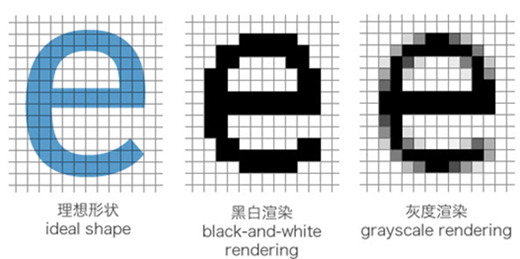##### 网站与APP开发中的字体设置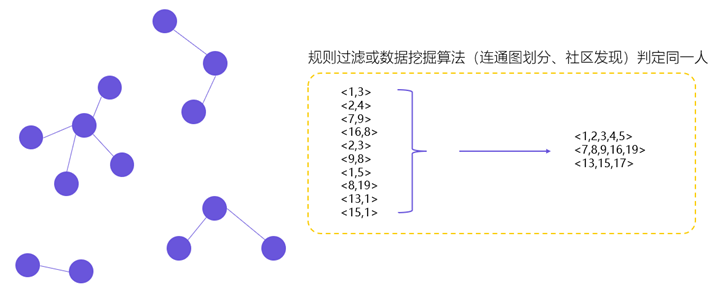##### 用户体系搭建之ID-Mapping